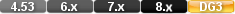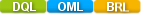Easy to Create, Easy to Change - Easy to use!## Log10Log10(NUMERICAL VALUE) Log10(3) Log10(MyNumberField)

The log10 function computes the common base 10 logarithm of a numeric value.

The numeric value in a Scientific function can be a constant value (as shown below), a variable, a field value, or an expression.

# Parameters

NUMERICAL VALUE

The number for which you want to compute the common base 10 logarithm for.

A numeric value.

# Examples

Examples

log10( 3)

Returns: 0.477121

log10( 0.5)

Returns: -0.301031

# log10

#### Type

Scientific Function

#### Purpose

The log10 function computes the common base 10 logarithm of a numeric value.

Syntax

log10( NUMERIC VALUE)

Returns

A numeric value.

Usage

The numeric value in a Scientific function can be a constant value (as shown below), a variable, a field value, or an expression.

Examples

log10( 3)

Returns: 0.477121

log10( 0.5)

Returns: -0.301031